Home » COALESCE Function DAX

# COALESCE Function DAX### COALESCE Function is a power Bi Dax Logical function that returns the first expression that does not evaluate to BLANK. It introduced in march 2020.

COALESCE Function can takes multiple arguments and returns the first argument that is not blank. If the first argument is evaluated to blank then It returns the value given by the expression in the second argument, and so on.

If all the arguments are evaluated to blank then COALESCE Function returns blank as well.

`COALESCE(<expression>, <expression>[, <expression>]…)`

expression is any DAX expression. It returns a scalar value.

Lets look at an example of COALESCE Function.

Here we have a dataset, as you can see below for Qty1 column all values are blank while Qty2 there is no blank values.

Item              Qty1     Qty2

 HP-NoteBook null 150 Samsung null 200 Lenevo null 400 Apple null 100 Acer null 40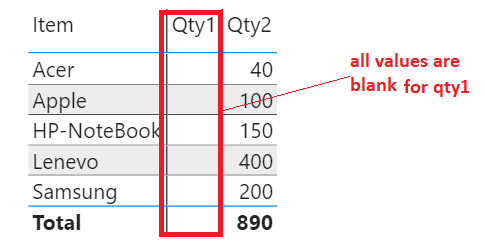For Qty1 column all values are given blank purposely to demonstrate the COALESCE function behaviour.

Suppose you have a requirement to display the total sum of quantity for an Items that is in Qty1 column and if total sum comes blank then display an alternate value that would be a total sum of quantity that is in Qty2 column.

Obviously, the total quantity sum for Qty1 column will be blank as all values are blank so in this case we would calculate the total quantity sum for Qty2 column.

Lets see, How COALESCE Function can be used for this requirement.

Here we have a measure Qty1_Sum, which calculates the sum of quantities for column Qty1.

`Qty1_Sum = SUM('Item'[Qty1])`The output of Measure Qty1_Sum is given below, It returns blank.Here we have one more measure that is Qty2_Sum, which calculates the sum of quantities for Qty2 column.

`Qty2_Sum = SUM('Item'[Qty2])`Lets see the output of Qty2_Sum measure as given below, It returns the total quantity sum for Qty2 that is 890.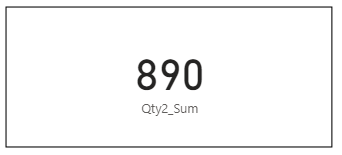As we have seen above both measures, Qty1_Sum measure returns blank while Qty2_Sum measure returns non blank value.

Lets create a Measure TotalQty which uses COALESCE function to check for first non blank value.

If first argument evaluates to blank then return the values from second argument , If it is also blank then returns blank.

In the first argument we have passed a measure Qty1_Sum and for second argument measure Qty2_Sum.

```TotalQty =
VAR Qty1 = [Qty1_Sum]
VAR Qty2 = [Qty2_Sum]
RETURN
COALESCE(Qty1, Qty2)```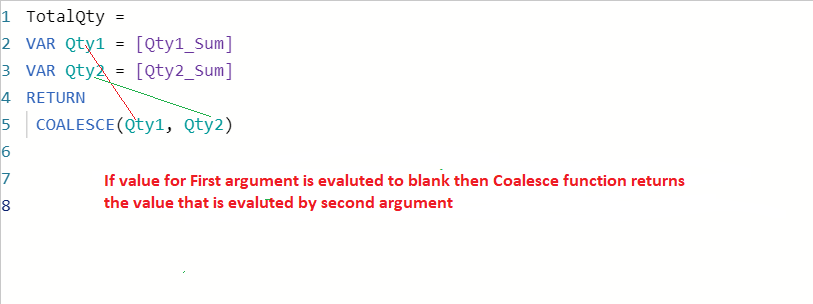Lets see the output of COALESCE Function, Drag the TotalQty measure into card visual.As you can see, it returns the output of measure Qty2_Sum that is a second argument in COALESCE Function as first arguments returned blank.

This is done for two argument values using COALESCE Function, in similar way you can also check for multiple arguments, if first and second argument returns blank value then returns the value from third argument that is non blank.

To check this in action, Lets create a measure that uses the COALESCE function and returns first non blank values among three given values for arguments that is blank , blank and 100.

`Get_NonBlank_Value = COALESCE(BLANK(),BLANK(),100)`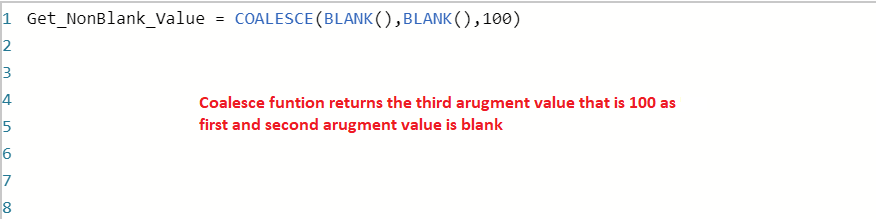Lets see the output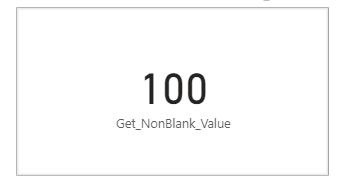You can see, it returns third argument value 100  that is non blank.This site uses Akismet to reduce spam. Learn how your comment data is processed.